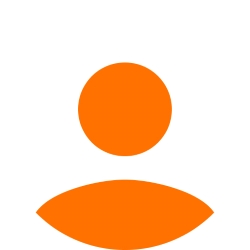gogodam93 25 Точки

Задачи за упражнение.

Здравейте :)

Споделяйте тук допълнителни задачи за упражнение, мисля че това би било полезно за всички нас !

Тагове:
10coaster 412 Точки

Ето няколко интересни примерчета... Давам и примерни решения на някои от тях. Някои са мои и до известна степен работят (т.е. никъде не съм включвала exception handling), но може да ги използвате за упътване и да направите по-добри програми. Успех! :)

1.
Write a program that checks if a number is even or odd. (give the value for the number variable from the code)
sln: http://pastebin.com/MjJdeS4P

2.
Write a program that checks if a number is divisible by 5 and 7.
sln: http://pastebin.com/8nFiHCre

3.
Write a program which calculates the area and the perimeter of a rectangle with given length and width.
sln: http://pastebin.com/HxCSHsGf

4.
Write a program which can do the operations bellow for a given four-digit number in abcd format (for example 2011):
- Calculates the sum of the four digits ( 2+0+1+1 = 4).
- Prints the digits in reverse order: dcba (for the example number the result should be 1102).
- Puts the last digit in first place: dabc (for the example number the result should be 1201).
- Switches the places of the second and the third digits: acbd (for the example number the result should be 2101).
sln: http://pastebin.com/nEB7Y8kp

5.
Write a program that prints all the numbers divisible by 3,4 and 9 within a given range. Arrange a menu to choose a divisor and use switch to cover all the cases.
sln: http://pastebin.com/bX8xTvez

6.
Write a program that checks if a given number is prime.
Algorithm 1 – calculate the integer M that is the closest integer to the square root of a given integer N. Using a loop from 2 to M check if N is divisible by any number within this range.
Algorithm 2 – calculate the integer M that is the closest integer to the half of a given number N. Using a loop from 2 to M check if N is divisible by any number within this range.
Arrange a menu for choosing between the algorithms.
sln: http://pastebin.com/BLG72Mar

7.
Write a program which converts to uppercase all the letters between every <up> and </up> tags in text entered by a user.
sln:
http://pastebin.com/BfUXks3h

8.
Write a program, which prints all sentences from some text, containing a particular word. Consider that all sentences are separated by
".".
sln: http://pastebin.com/vX2nH8Vi

9.
Write a program that calculates the roots of a
quadraticequationentered in the following format  ax^2+bx+c=0.
sln: http://pastebin.com/BMCzM81X

10.
Write a program that counts how many times a chosen day of week is repeated between two dates.

11. (this is my personal favorite, it requires you to look for some more info on user IDs :)
Write a program that from a given Personal ID can extract someone’s gender, age, date of birth and number of days until their next birthday.
sln:
http://pastebin.com/GVKAFERc

12.
Write a program that creates an array of 20 int objects  and initializes each of them with a value equal to the product of its index and 5. Then print all the elements of the array in the console.
* Declaring an array
: int[] numbers = new int;
* Iterating through the elements
: for this task it’s suitable to use the for loop and use its counter variable as an element index

13.
Write a program that reads two arrays from the console and checks if they are the same (compare the values of the elements in corresponding positions).
sln:
http://pastebin.com/8UfG1Mb

14.
Write a program that reads two int arrays from the console and checks if they have equal elements (in any position). Put the equals elements in another array and print the highest value of them.
* Resizing an array: Array.Resize(ref
arrayName, newsize)
* Sorting: Array.Sort
(arrayName)
sln: http://pastebin.com/AT70rsjg

15.
Write a program that compares two char arrays lexicographically and tells which one comes first.
sln:
http://pastebin.com/RJxAqd6z

16.
Write a program which finds the longest sequence of equal  elements in an array.
Example: {2, 1, 1, 2, 3, 3, 2, 2, 2, 1} --> {2, 2, 2}.

17.
Write a program which finds the longest sequence of consecutive numbers in an array.
Example: {3, 2, 3, 4, 2, 2, 4} --> {2, 3, 4}.

18.
Write a program which finds the longest sequence of elements in arithmetic progression. The elements may not be ordered in the array.
Example
: {9, 6, 2, 7, 4, 7, 6, 5, 8, 4} --> {2, 4, 6, 8}.

19.
Write a program that reads two int numbers from the console N and K (K<N), and an array of N elements. Find the sequence of K elements that have the highest sum.
_____________________________

И още нещо за упражнение на работа с отделни класове, наследяване и т.н.

http://dox.bg/files/dw?a=38ed209940

3Anichka 93 Точки
Направо започвай да качваш имплементациите :)
0KolevT 0 Точки

Случайно да имаш работещо решение на 18-ти проблем?

0coaster 412 Точки
Готово :)
0